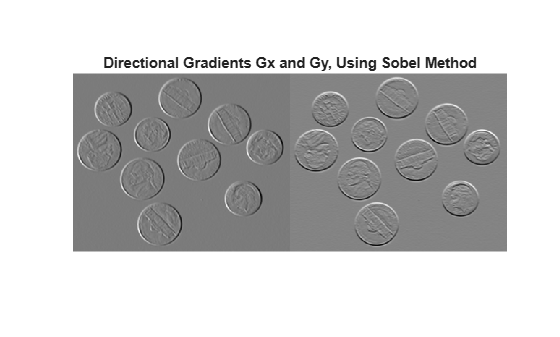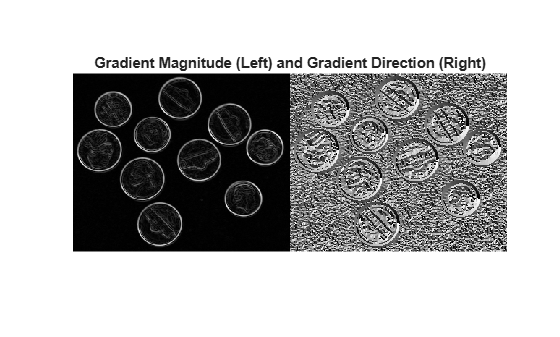Find gradient magnitude and direction of 2-D image

## Syntax

``````[Gmag,Gdir] = imgradient(I)``````
``````[Gmag,Gdir] = imgradient(I,method)``````
``````[Gmag,Gdir] = imgradient(Gx,Gy)``````

## Description

``````[Gmag,Gdir] = imgradient(I)``` returns the gradient magnitude, `Gmag`, and the gradient direction, `Gdir`, of the 2-D grayscale or binary image `I`. ```

example

``````[Gmag,Gdir] = imgradient(I,method)``` returns the gradient magnitude and direction using the specified `method`.```

example

``````[Gmag,Gdir] = imgradient(Gx,Gy)``` returns the gradient magnitude and direction from the directional gradients `Gx` and `Gy` in the x and y directions, respectively.```

## Examples

collapse all

`I = imread('coins.png');`

`[Gmag, Gdir] = imgradient(I,'prewitt');`

Display the gradient magnitude and direction.

```figure imshowpair(Gmag, Gdir, 'montage'); title('Gradient Magnitude, Gmag (left), and Gradient Direction, Gdir (right), using Prewitt method')````I = imread('coins.png');`

Calculate the x- and y-directional gradients. By default, `imgradientxy` uses the Sobel gradient operator.

`[Gx,Gy] = imgradientxy(I);`

```imshowpair(Gx,Gy,'montage') title('Directional Gradients Gx and Gy, Using Sobel Method')````[Gmag,Gdir] = imgradient(Gx,Gy);`

Display the gradient magnitude and direction.

```imshowpair(Gmag,Gdir,'montage') title('Gradient Magnitude (Left) and Gradient Direction (Right)')```## Input Arguments

collapse all

Input image, specified as a 2-D grayscale or 2-D binary image.

Data Types: `single` | `double` | `int8` | `int32` | `uint8` | `uint16` | `uint32` | `logical`

Gradient operator, specified as one of the following values.

MethodDescription
`"sobel"`

Sobel gradient operator. The gradient of a pixel is a weighted sum of pixels in the 3-by-3 neighborhood. For gradients in the vertical (y) direction, the weights are:

```[ 1 2 1 0 0 0 -1 -2 -1 ]```
In the x direction, the weights are transposed.

`"prewitt"`

Prewitt gradient operator. The gradient of a pixel is a weighted sum of pixels in the 3-by-3 neighborhood. For gradients in the vertical (y) direction, the weights are:

```[ 1 1 1 0 0 0 -1 -1 -1 ]```
In the x direction, the weights are transposed.

`"central"`

Central difference gradient. The gradient of a pixel is a weighted difference of neighboring pixels. In the y direction, ```dI/dy = (I(y+1) - I(y-1))/2```.

`"intermediate"`

Intermediate difference gradient. The gradient of a pixel is the difference between an adjacent pixel and the current pixel. In the y direction, ```dI/dy = I(y+1) - I(y)```.

`"roberts"`

Roberts gradient operator. The gradient of a pixel is the difference between diagonally adjacent pixels. For gradients in one direction, the weights are:

```[ 1 0 0 -1 ]```
In the orthogonal direction, the weights are flipped along the vertical axis.

Data Types: `char` | `string`

Horizontal gradient, specified as a numeric matrix. The horizontal (x) axis points in the direction of increasing column subscripts. You can use the `imgradientxy` function to calculate `Gx`.

Data Types: `single` | `double` | `int8` | `int32` | `uint8` | `uint16` | `uint32`

Vertical gradient, specified as a numeric matrix of the same size as `Gx`. The vertical (y) axis points in the direction of increasing row subscripts. You can use the `imgradientxy` function to calculate `Gy`.

Data Types: `single` | `double` | `int8` | `int32` | `uint8` | `uint16` | `uint32`

## Output Arguments

collapse all

Gradient magnitude, returned as a numeric matrix of the same size as image `I` or the directional gradients `Gx` and `Gy`. `Gmag` is of class `double`, unless the input image or directional gradients are of data type `single`, in which case it is of data type `single`.

Data Types: `double` | `single`

Gradient direction, returned as a numeric matrix of the same size as gradient magnitude `Gmag`. `Gdir` contains angles in degrees within the range [-180, 180] measured counterclockwise from the positive x-axis. (The x-axis points in the direction of increasing column subscripts.) `Gdir` is of class `double`, unless the input image `I` or directional gradients are of data type `single`, in which case it is of data type `single`.

Data Types: `double` | `single`

## Tips

• When applying the gradient operator at the boundaries of the image, values outside the bounds of the image are assumed to equal the nearest image border value. This is similar to the `"replicate"` boundary option in `imfilter`.

## Algorithms

The algorithmic approach taken in `imgradient` for each of the listed gradient methods is to first compute directional gradients, `Gx` and `Gy`, in the x and y directions, respectively. The horizontal (x) axis points in the direction of increasing column subscripts. The vertical (y) axis points in the direction of increasing row subscripts. The gradient magnitude and direction are then computed from their orthogonal components `Gx` and `Gy`.

`imgradient` does not normalize the gradient output. If the range of the gradient output image has to match the range of the input image, consider normalizing the gradient image, depending on the `method` argument used. For example, with a Sobel kernel, the normalization factor is 1/8, for Prewitt, it is 1/6, and for Roberts it is 1/2.

## Version History

Introduced in R2012b

expand all